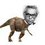# Proof Contest Day 2 (Follow Up problem)

The previous problem dealt with proving that the sum of heights remains constant. However , in this follow up problem we would find the exact value of the sum of heights for an even sided polygon.

PROBLEM:

Let $P$ be any point in the interior of a regular polygon of $2n$ sides. Perpendiculars $PA_1 \ , \ PA_2 \ , \ PA_3 \ , \ \dots \ , \ PA_{2n}$ are drawn to the sides of the polygon. Show that: $\displaystyle\sum_{i=1}^{2n} PA_{i} = 2nr$ where $r$ is the radius of inscribed circle of polygon.

Also show that $\displaystyle\sum_{i=1}^{n} PA_{2i-1} =\sum_{j=1}^{n} PA_{2j} =nr$

###### This problem is not originalNote by Nihar Mahajan
5 years, 6 months ago

This discussion board is a place to discuss our Daily Challenges and the math and science related to those challenges. Explanations are more than just a solution — they should explain the steps and thinking strategies that you used to obtain the solution. Comments should further the discussion of math and science.

When posting on Brilliant:

• Use the emojis to react to an explanation, whether you're congratulating a job well done , or just really confused .
• Ask specific questions about the challenge or the steps in somebody's explanation. Well-posed questions can add a lot to the discussion, but posting "I don't understand!" doesn't help anyone.
• Try to contribute something new to the discussion, whether it is an extension, generalization or other idea related to the challenge.

MarkdownAppears as
*italics* or _italics_ italics
**bold** or __bold__ bold
- bulleted- list
• bulleted
• list
1. numbered2. list
1. numbered
2. list
Note: you must add a full line of space before and after lists for them to show up correctly
paragraph 1paragraph 2

paragraph 1

paragraph 2

[example link](https://brilliant.org)example link
> This is a quote
This is a quote
    # I indented these lines
# 4 spaces, and now they show
# up as a code block.

print "hello world"
# I indented these lines
# 4 spaces, and now they show
# up as a code block.

print "hello world"
MathAppears as
Remember to wrap math in $$ ... $$ or $ ... $ to ensure proper formatting.
2 \times 3 $2 \times 3$
2^{34} $2^{34}$
a_{i-1} $a_{i-1}$
\frac{2}{3} $\frac{2}{3}$
\sqrt{2} $\sqrt{2}$
\sum_{i=1}^3 $\sum_{i=1}^3$
\sin \theta $\sin \theta$
\boxed{123} $\boxed{123}$

Sort by:

The first one can be pretty easily proved using the fact that the radius of the inscribed circle is the height of the triangle.The second one can be proved using the fact that since the polygpn has an even number of sides the two perpendiculars,$PA_{i},PA_{i+n}$ form a straight line,from here we easily get that,$PA_{i}+PA_{i+n}=PA_{k}+PA_{k+n}$,now just put values,$1,3,5...(n-1)$ for i and the rest for k and then add to get the result,i am writing this on phone so cant explain very clearly.

- 5 years, 6 months ago

Exactly! Nice use of the fact that since the polygon has an even number of sides the two perpendiculars, $PA_i , PA_{i+n}$ form a straight line.

- 5 years, 6 months ago

- 5 years, 6 months ago

We know that the sum of the distances of the perpendiculars from the interior point is always constant. (As proved earlier). So we know that $PA_1+...+PA_{2n}=k$ a constant.

Now the incentre of the polygon is also such a point so the sum of the perpendiculars from it will be the sum of $2n$ radii. So we get that $PA_1+PA_2+...+PA_{2n}=2nr=k$

- 5 years, 6 months ago

Can we directly prove the last thing?

- 5 years, 6 months ago

Yes. Then the main problem becomes obvious from there.

- 5 years, 6 months ago